﻿ 基于EEMD-ARIMA模型的气温预测研究

# 基于EEMD-ARIMA模型的气温预测研究Prediction of Temperature Based on EEMD-ARIMA Model

Abstract: The average annual temperature data are modeled and predicted by ensemble empirical mode decomposition (EEMD) and autoregressive integrated moving (ARIMA) model. First, this paper performs the noise processing on the average annual temperature, and performs empirical mode decomposition (EMD) to make the components smoothed. Then, each component is predicted by ARIMA model. Finally, the component prediction results are added to obtain the final predicted value of the average annual temperature. By comparison, it is found that the prediction results of the EEMD-ARIMA model are more accurate than the prediction results of the EMD-ARIMA model and the ARIMA model.

1. 引言

1998年，N.E. Huang提出了一种新的信号分析方法——经验模态分解(EMD) 。该方法在处理非平稳非线性的数据上具有很大的优势，一经提出后，就在不同的工程领域得到了迅速有效的应用，例如在海洋、大气、天体观测资料与地震记录分析、机械故障诊断 、密频动力系统的阻尼识别以及大型土木工程结构的模态参数识别等方面。但是，如果数据出现模态混叠现象，该方法的性能会出现大幅度下降的情况，因此N.E. Huang为了改进EMD的不足，提出了EEMD方法 ，用以解决EMD中的模态混叠问题，该方法在气候、工程等领域有较多的应用。

2. EEMD基本原理

1998年N.E. Huang  提出了EMD方法分析非线性、非平稳序列，此方法的基本思想是把一个频率不规则的波转化为多个单一频率的波(本征模态函数IMF)和残波的形式。但有时EMD会出现不同程度的模态混叠现象，为了解决这个现象，WU等  提出了集合经验模态分解方法(EEMD)。EEMD是一种基于EMD，用噪声进行辅助的数据分析方法，EEMD的核心思想是将白噪声加入原始信号中进行多次分解，把分解多次的本征模态函数(IMF)和趋势项取均值，该值为最终的IMF分量和rs余项。利用EEMD分解原始信号的具体步骤如下：

1) 添加白噪声的次数(即试验总次数) ${n}_{e}$ 和幅值系数 $\epsilon$

2) 将白噪声加入原始信号 $x\left(t\right)$ 中， $w\left(t\right)$ 是第i次加入的白噪声序列，可得到信号 ${X}^{\prime }\left(t\right)$

${X}^{\prime }\left(t\right)=x\left(t\right)+\epsilon \ast w\left(t\right)$ (1)

3) 找出要分解的时间序列 ${X}^{\prime }\left(t\right)$ 所有的局部最大值点和最小值点，利用三次样条函数的方法拟合 ${X}^{\prime }\left(t\right)$ 所有的最大值点和最小值点，构成上包络线和下包络线。

4) ${b}_{1}\left(t\right)$ 为上下包络线的平均值。将信号 ${X}^{\prime }\left(t\right)$${b}_{1}\left(t\right)$ 相减，得到新序列：

${{X}^{″}}_{1}\left(t\right)={X}^{\prime }\left(t\right)-{b}_{1}\left(t\right)$ (2)

5) 判断 ${{X}^{″}}_{1}\left(t\right)$ 是否满足IMF分量的条件，如果满足，则 ${{X}^{″}}_{1}\left(t\right)$ 是筛选出的第一个IMF分量为 ${\text{IMF}}_{1}$ ；如果不满足， ${{X}^{″}}_{1}\left(t\right)$ 将作为新的原始序列重新回到步骤(3)和步骤(4)继续进行筛选，直到满足IMF分量的条件。

6) 把 ${\text{IMF}}_{1}$ 从信号 ${X}^{\prime }\left(t\right)$ 中减去得到 ${x}_{1}$，如式(3)所示。把 ${x}_{1}$ 作为要分解的新信号，重复步骤(3)至步骤(6) n次，直到 ${x}_{n}$ 或者 ${\text{IMF}}_{n}$ 小于给定的数值或 ${x}_{n}$ 为单调函数，即可结束分解。

${x}_{1}={X}^{\prime }\left(t\right)-{\text{IMF}}_{1}$ (3)

7) 用SD (限值标准差)判断筛选是否终止。当SD小于阈值 $\alpha$ 时，筛选结束。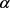的一般取值范围是0.2~0.3。 ${X}^{\prime }\left(t\right)$ 经步骤(3)至步骤(7)处理后即可得到不同尺度的IMF和余项。

$\text{SD}=\frac{\underset{t=0}{\overset{T}{\sum }}{\left({{X}^{″}}_{k-1}^{2}\left(t\right)-{{X}^{″}}_{k}^{2}\left(t\right)\right)}^{2}}{\underset{t=0}{\overset{T}{\sum }}{{X}^{″}}_{k-1}^{2}\left(t\right)}$ (4)

8) 利用白噪声频谱的均值为零，将 ${n}_{e}$ 次分解得到的各IMF进行均值处理，得到EEMD分解后最终的IMF分量 ${c}_{n}\left(t\right)$ 和趋势项 $r\left(t\right)$

${c}_{s}\left(t\right)=\frac{1}{{n}_{e}}\underset{i=1}{\overset{{n}_{e}}{\sum }}{\text{IMF}}_{is}\left(t\right)$ (5)

$r\left(t\right)=\frac{1}{{n}_{e}}\underset{i=1}{\overset{{n}_{e}}{\sum }}{r}_{i}\left(t\right)$ (6)

9) 最后 $x\left(t\right)$ 的分解结果为：

$x\left(t\right)=\underset{s=1}{\overset{n}{\sum }}{c}_{s}\left(t\right)+r\left(t\right)$ (7)

3. EEMD-ARIMA预测模型基本原理

3.1. ARIMA模型

ARIMA(p, d, q)是最常用的时间序列模型  ，其中AR是自回归过程，参数p是其自回归项项数；MA是移动平均过程，参数q是其移动平均项数；参数d是使研究对象的时间序列达到平稳状态的差分次数。ARIMA(p, d, q)模型结构为：

$\left\{\begin{array}{l}\Phi \left(B\right){\nabla }^{d}{x}_{t}=\Theta \left(B\right){\epsilon }_{t}\\ E\left({\epsilon }_{t}\right)=0,Var\left({\epsilon }_{t}\right)={\sigma }_{t}^{2},E\left({\epsilon }_{t}{\epsilon }_{s}\right)=0,s\ne t\\ E\left({x}_{s}{\epsilon }_{t}\right)=0,\text{}\forall s (8)

3.2. EEMD-ARIMA模型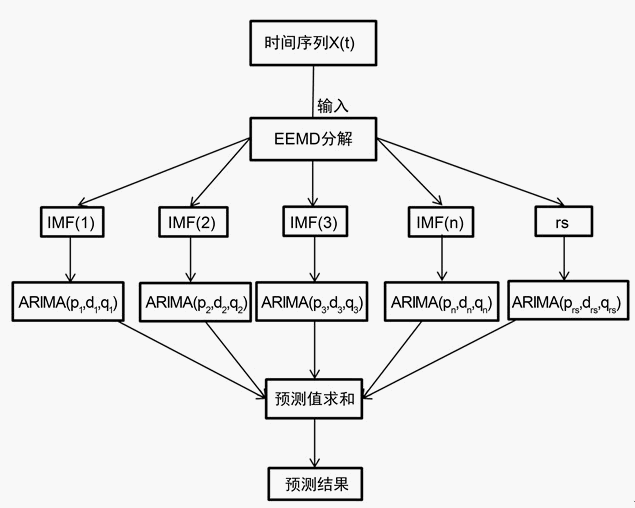Figure 1. Flow chart of EEMD-ARIMA prediction model

EEMD-ARIMA预测模型的具体步骤如下：

1) 首先，将原始信号 $X\left(t\right)$ 输入EEMD中进行分解， $X\left(t\right)$ 会被分解成若干个IMF和余项rs。

2) 其次，因为通过EEMD分解得到的若干个IMF和rs之间相互独立，所以，对每一个IMF和rs进行ARIMA建模，并求出相应的预测值。

3) 最后，将所求得的每个分量的预测值相加，其和为最终的气温预测数据。

4. 模型应用

4.1. EMD与EEMD去噪效果分析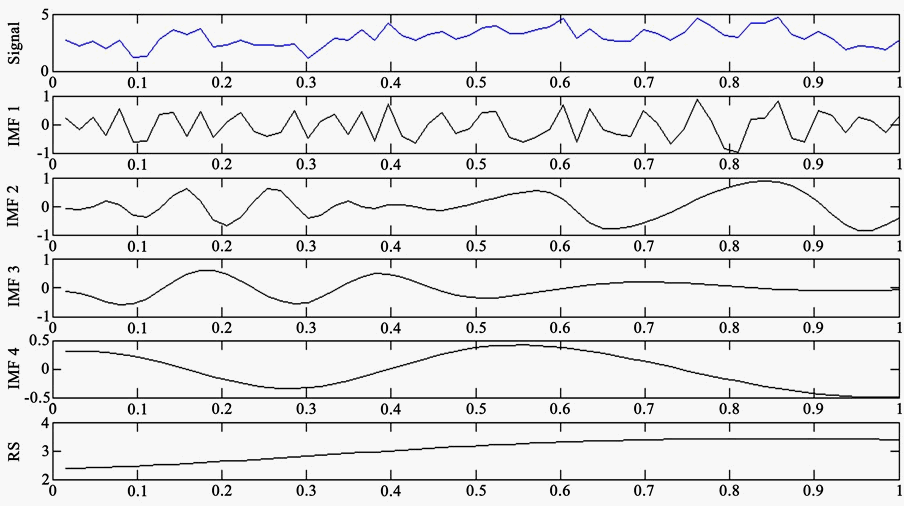Figure 2. EMD component of annual average temperature in Shenyang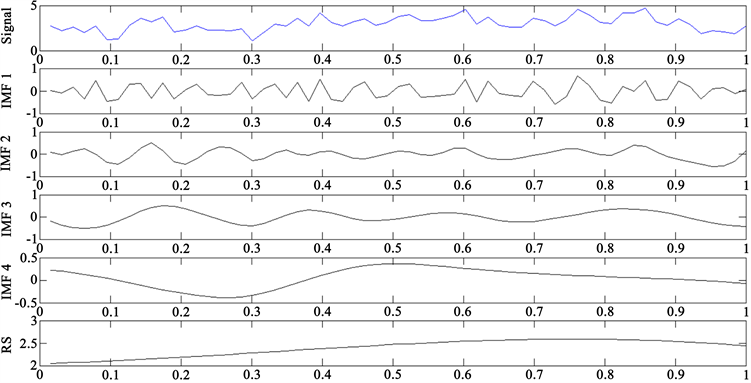Figure 3. EEMD component of annual average temperature in Shenyang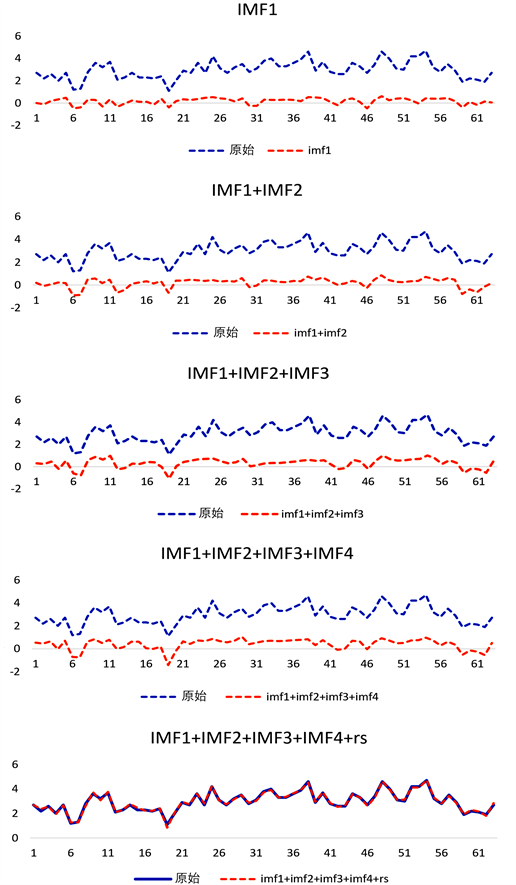Figure 4. Sequence reconstruction of EEMD decomposition components

$\text{MSE}=\frac{{\sum }_{t=1}^{n}{|x\left(t\right)-{x}^{\prime }\left(t\right)|}^{2}}{n}$ (9)Table 1. MSE calculation value

4.2. 年均气温预测结果及分析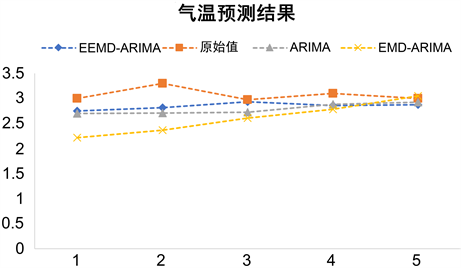Figure 5. Temperature prediction chart of three models

$\text{MAE}=\frac{1}{n}\underset{t=1}{\overset{n}{\sum }}|x\left(t\right)-{x}^{\prime }\left(t\right)|$ (10)Table 2. Calculated value of mean absolute error (MAE)

5. 结论

 Abuella, M. and Chowdhury, B, (2015) Solar Power Probabilistic Forecasting by Using Multiple Linear Regression Analysis. SoutheastCon 2015, Fort Lauderdale, 9-12 April 2015, 1-5.
https://doi.org/10.1109/SECON.2015.7132869

 Pratiher, S., Mukhopadhyay, S., Barman, R., et al. (2016) Recurrence Quantification & ARIMA Based Forecasting of Rainfall-Temperature Dynamics. 2016 International Conference on Signal Processing and Communication (ICSC), Noida, 26-28 December 2016, 490-495.
https://doi.org/10.1109/ICSPCom.2016.7980630

 Huang, C.Y., Liu, Y.W., Tzeng, W.C., et al. (2011) Short Term Wind Speed Predictions by Using the Grey Prediction Model Based Forecast Method. 2011 IEEE Green Technologies Conference (IEEE-Green), Baton Rouge, 14-15 April 2011, 1-5.
https://doi.org/10.1109/GREEN.2011.5754856

 陶晔, 杜景林. 基于随机森林的长短期记忆网络气温预测[J]. 计算机工程与设计, 2019, 40(3): 737-743.

 黄建风, 陆文聪. 基于小波-NAR神经网络的气象要素时间序列预测与天气指数彩虹期权估值[J]. 系统工程理论与实践, 2016, 36(5): 1146-1155.

 王定成, 曹智丽, 陈北京, 等. 日气温多元时间序列局部支持向量回归预测[J]. 系统仿真学报, 2016, 28(3): 654-660.

 Huang, N.E., Shen, Z., Long, S.R., et al. (1988) The Empirical Mode Decomposition and the Hibert Spectrum for Nonlinear and Nonstationary Time Series Analysis. Proceedings of the Royal Society A: Mathematical, Physical and Engineering Sciences, 454, 903-995.
https://doi.org/10.1098/rspa.1998.0193

 葛兴来, 邹丹. 基于多层降噪技术及Hilbert变换的轴承故障诊断方法[J/OL]. 电机与控报, 1-12.
http://kns.cnki.net/kcms/detail/23.1408.TM.20191226.1452.006.html, 2020-03-06.

 Wu, Z.H. and Huang, N.E. (2008) Ensemble Empirical Mode Decomposition: A Noise-Assisted Data Analysis Method. Advances in Adaptive Data Analysis, 1, 1-41.
https://doi.org/10.1142/S1793536909000047

 麦鸿坤, 肖坚红, 吴熙辰, 等. 基于R语言的负荷预测ARIMA模型并行化研究[J]. 电网技术, 2015, 39(11): 3216-3220.

 Huang, S.J. and Shih, K.R. (2003) Short-Term Load Forecasting via ARMA Model Identification Including Non-Gaussian Process Considerations. IEEE Transactions on Power Systems, 18, 673-679.
https://doi.org/10.1109/TPWRS.2003.811010

Top